# Wilcox Solar Observatory Magnetograms

## #8000 - 8009, 2004.07.03 - 2004.07.12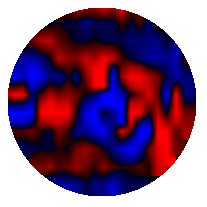```WSO Magnetogram sn=8000	 observed at 2004:07:03_22h:15m:59s
Central Meridian Carrington Longitude CT2018:244
Sky = 0 (5 worst)
trouble=00004000 NOTE:
12 intensity variance exceeds 5.0
```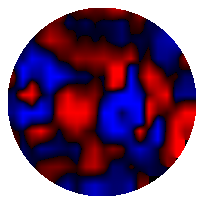```WSO Magnetogram sn=8001	 observed at 2004:07:04_17h:53m:27s
Central Meridian Carrington Longitude CT2018:233
Sky = 0 (5 worst)
trouble=00004000 NOTE:
12 intensity variance exceeds 5.0
```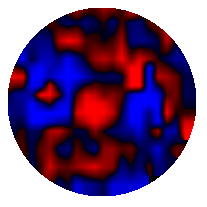```WSO Magnetogram sn=8002	 observed at 2004:07:05_17h:22m:31s
Central Meridian Carrington Longitude CT2018:220
Sky = 0 (5 worst)
trouble=00004000 NOTE:
12 intensity variance exceeds 5.0
```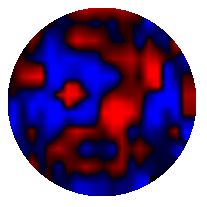```WSO Magnetogram sn=8003	 observed at 2004:07:06_21h:25m:19s
Central Meridian Carrington Longitude CT2018:205
Sky = 0 (5 worst)
trouble=00004000 NOTE:
12 intensity variance exceeds 5.0
```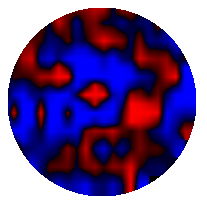```WSO Magnetogram sn=8004	 observed at 2004:07:07_23h:20m:54s
Central Meridian Carrington Longitude CT2018:190
Sky = 0 (5 worst)
trouble=00004000 NOTE:
12 intensity variance exceeds 5.0
```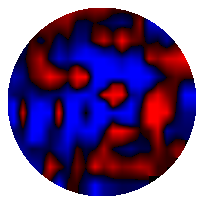```WSO Magnetogram sn=8005	 observed at 2004:07:08_20h:39m:39s
Central Meridian Carrington Longitude CT2018:179
Sky = 0 (5 worst)
trouble=00004000 NOTE:
12 intensity variance exceeds 5.0
```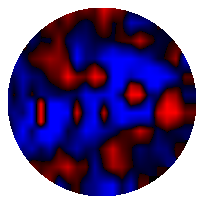```WSO Magnetogram sn=8006	 observed at 2004:07:09_18h:43m:44s
Central Meridian Carrington Longitude CT2018:166
Sky = 1 (5 worst)
trouble=00004000 NOTE:
12 intensity variance exceeds 5.0
```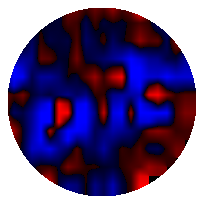```WSO Magnetogram sn=8007	 observed at 2004:07:10_22h:56m:08s
Central Meridian Carrington Longitude CT2018:151
Sky = 0 (5 worst)
trouble=00004000 NOTE:
12 intensity variance exceeds 5.0
```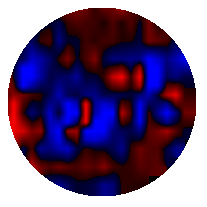```WSO Magnetogram sn=8008	 observed at 2004:07:11_23h:42m:51s
Central Meridian Carrington Longitude CT2018:137
Sky = 0 (5 worst)
trouble=00004000 NOTE:
12 intensity variance exceeds 5.0
```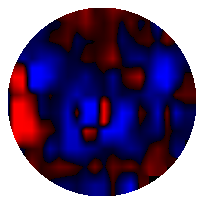```WSO Magnetogram sn=8009	 observed at 2004:07:12_20h:13m:24s
Central Meridian Carrington Longitude CT2018:126
Sky = 0 (5 worst)
trouble=00004000 NOTE:
12 intensity variance exceeds 5.0
```